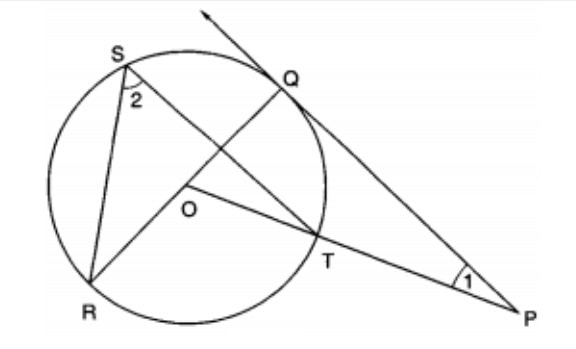Q)

In figure, PQ is a tangent from an external point P to a circle with centre O and OP cuts the circle at T and QOR is a
diameter. If ∠POR = 130° and S is a point on the circle, find ∠1 +∠2Ans:

In the given diagram, it is given that: ∠ POR = 1300

We know that the  Angle subtended at the center by same arc is half than that of center,

∴ ∠TOR = 2 ∠2

∴ 1300 = 2 ∠2

∴  ∠2 = 65…… equation (1)

∵ ∠ROT = 1300

∴  ∠QOT = 180 – 130 = 50

Also we can see that, ∠POQ = ∠QOT = 50.….. equation (2)

We know that the angle between the radius and the tangent is 900 at the point of contact,

therefore ∠ PQO = 900.….. equation (3)

In Δ POQ,  ∠1 + ∠POQ + ∠PQO = 1800

By putting values from equations (2) & (3), we get:

∠1 + ∠POQ + ∠PQO = 1800

∴ ∠1 + 500 + 900 = 1800

∴ ∠1  = 400 .…. equation (4)

By adding equations (1) and (4), we get:

∠ 1 + ∠ 2 = 400  + 65

∠ 1 + ∠ 2 = 1050

Scroll to Top